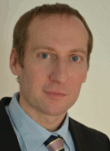Robert Kosik Dipl.-Ing. Dr.techn. Publications### Biography

Robert Kosik was born in Eisenstadt, Austria, in 1969. He studied technical mathematics at the Technische Universität Wien, where he received the degree of Diplomingenieur in 1996. In 1998 he joined the Institute for Microelectronics at the Technische Universität Wien and received his PhD degree in 2004. His scientific interests include partial differential equations for transport modeling and data analysis in reliability.

## Non-spatial Open Boundary Conditions for the Wigner Equation

The Wigner equation describing stationary quantum transport has a singularity at zero momentum (k=0). We have previously proposed a revised approach which explicitly includes the point k=0 in the mesh. This approach yields two equations for k=0 and, thus, an overdetermined equation system. The sigma equation is the inverse Fourier transform of the Wigner equation. The two equations for k=0 are related to double homogeneous boundary conditions for the sigma equation in the non-spatial coordinate. The natural way to incorporate double homogeneous boundary conditions is by employing an absorbing potential layer. This has the effect of damping down the solution near the non-spatial boundary.

We have introduced an alternative formulation of the open boundary conditions based on a symmetric treatment of the spatial and non-spatial coordinates of the sigma equation. We apply inflow-type boundary conditions also at the non-spatial boundaries. {?}For this we use the Fourier transform of the characteristic function in the spatial coordinate which is denoted ambiguity function.{?} We impose the condition that the flow into the domain at the non-spatial boundaries is zero. The developed numerical schemes have been implemented and tested. Both schemes closely reproduce results from the quantum transmitting boundary method. This work clarifies the notion of open boundary conditions for the Wigner and the characteristic Neumann equation, and demonstrates its numerical robustness.

A peculiarity is that the zero bias case in the Wigner equation is special, as it allows for (physically sound) distributional parts in the solution. These distributional parts are a pure quantum effect with no classical analogue, as they stem from the long range correlation between reflected and outgoing parts of a mode. Our method excludes distributional parts. However, distributional parts are contained in the distribution function stemming from the Wigner transform of the solution to the corresponding Schrödinger problem.

Below we compare simulation results from the quantum transmitting boundary method with results from the sigma equation with no-inflow boundary conditions. We calculate the particle density for a resonant tunneling diode at different biases. For zero bias, we actually see a small deviation in the density at the center of the well, but in this case the fit between the two methods is still good.Fig. 1: Comparison of particle density in the active region of a resonant tunneling diode at zero bias. The results from both methods show a small deviation at the center of the well.Fig. 2: Comparison of particle density in the active region of a resonant tunneling diode at a bias of 0.3 V. The results from both methods fit almost perfectly.